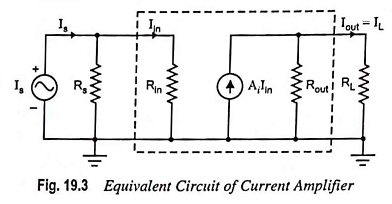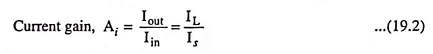## Current Amplifier – Definition and Equivalent Circuit:

The input signal in a current amplifier is essentially a current, and thus the signal source is most conveniently represented by its Norton equivalent (Fig. 19.3). The output quantity of interest is current. The current amplifiers is essentially a current-controlled current source.The equivalent circuit of a current amplifier is depicted in Fig. 19.3. In the given circuit if the input resistance Rin << Rs then Iin ≈ Is and if RL << Rout then IL = Iout = AiIinAn ideal current amplifiers should have zero input resistance and infinite output resistance.

An ideal current amplifiers is defined as an amplifier which provides an output current proportional to the signal current, and proportionality constant is independent of magnitudes of source and load resistances (Rs and RL respectively).

Scroll to Top